Download Presentation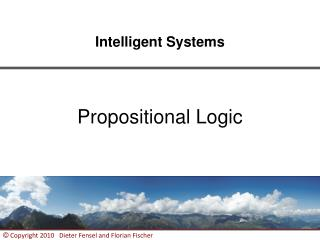Intelligent Systems

# Intelligent Systems - PowerPoint PPT Presentation

Intelligent Systems. Propositional Logic. Where are we?. Outline. Motivation Technical Solution Syntax Semantics Inference Illustration by a Larger Example Extensions Summary References. MOTIVATION. 4. Logic and Deduction. Logic is used to formalize deductionI am the owner, or an agent authorized to act on behalf of the owner, of the copyrighted work described.
Download Presentation## Intelligent Systems

An Image/Link below is provided (as is) to download presentation

Download Policy: Content on the Website is provided to you AS IS for your information and personal use and may not be sold / licensed / shared on other websites without getting consent from its author.While downloading, if for some reason you are not able to download a presentation, the publisher may have deleted the file from their server.

- - - - - - - - - - - - - - - - - - - - - - - - - - E N D - - - - - - - - - - - - - - - - - - - - - - - - - -
Presentation Transcript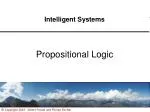### Intelligent Systems

Propositional Logic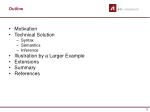Outline
• Motivation
• Technical Solution
• Syntax
• Semantics
• Inference
• Illustration by a Larger Example
• Extensions
• Summary
• References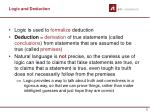Logic and Deduction
• Logic is used to formalize deduction
• Deduction = derivation of true statements (called conclusions) from statements that are assumed to be true (called premises)
• Natural language is not precise, so the careless use of logic can lead to claims that false statements are true, or to claims that a statement is true, even tough its truth does not necessarily follow from the premises

=> Logic provides a way to talk about truth and correctness in a rigorous way, so that we can prove things, rather than make intelligent guesses and just hope they are correct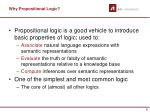Why Propositional Logic?
• Propositional logic is a good vehicle to introduce basic properties of logic; used to:
• Associate natural language expressions with semantic representations
• Evaluate the truth or falsity of semantic representations relative to a knowledge base
• Compute inferences over semantic representations
• One of the simplest and most common logic
• The core of (almost) all other logics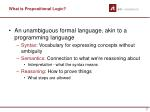What is Propositional Logic?
• An unambiguous formal language, akin to a programming language
• Syntax: Vocabulary for expressing concepts without ambiguity
• Semantics: Connection to what we're reasoning about
• Interpretation - what the syntax means
• Reasoning: How to prove things
• What steps are allowed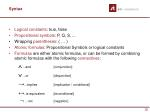Syntax
• Logical constants: true, false
• Propositional symbols: P, Q, S, ...
• Wrapping parentheses: ( … )
• Atomic formulas: Propositional Symbols or logical constants
• Formulas are either atomic formulas, or can be formed by combining atomic formulas with the following connectives:

...and [conjunction]

...or [disjunction]

→...implies [implication / conditional]

↔..is equivalent [biconditional]

...not [negation]Syntax (cont’)
• A sentence (well formed formula) is defined as follows:
• A symbol is a sentence
• If S is a sentence, then S is a sentence
• If S is a sentence, then (S) is a sentence
• If S and T are sentences, then (S  T), (S  T), (S  T), and (S ↔ T) are sentences
• A sentence results from a finite number of applications of the above rulesSyntax – BNF Grammar

Sentence  AtomicSentence | ComplexSentence

AtomicSentence  True | False | P | Q | R | ...

ComplexSentence  (Sentence )

| Sentence Connective Sentence

|  Sentence

Connective   |  |→|↔

Ambiguities are resolved through precedence   →↔or parentheses

e.g.  P  Q  R  S is equivalent to ( P)  (Q  R))  SSyntax – Examples
•  p Ù  q
• (p Ù q)
• (p Ù q) Ú r
• p Ù q Ù r
• ((( p) Ù q) ® r) « (( r) Ú p)
• ( (p Ú q) ® q) ® r
• (( p) Ú ( q)) « ( r)
• Etc.
• P means “It is hot.”
• Q means “It is humid.”
• R means “It is raining.”
• (P  Q)  R

“If it is hot and humid, then it is raining”

• Q  P

“If it is humid, then it is hot”Semantics
• Interpretations
• Equivalence
• Substitution
• Models and Satisfiability
• Validity
• Logical Consequence (Entailment)
• TheorySemantics – Some Informal Definitions
• Given the truth values of all symbols in a sentence, it can be “evaluated” to determine its truth value(True or False)
• A model for a KB is a “possible world” (assignment of truth values to propositional symbols) in which each sentence in the KB is True
• A valid sentenceor tautology is a sentence that is True under all interpretations, no matter what the world is actually like or how the semantics are defined (example: “It’s raining or it’s not raining”)
• An inconsistent sentenceor contradiction is a sentence that is False under all interpretations (the world is never like what it describes, as in “It’s raining and it’s not raining”)
• P entails Q, written P ⊧ Q, means that whenever P is True, so is Q; in other words, all models of P are also models of QInterpretations
• In propositional logic, truth values are assigned to the atoms of a formula in order to evaluate the truth value of the formula
• An assignment is a function

v : P → {T,F}

v assigns a truth value to any atom in a given formula (P is the set of all propositional letters, i.e. atoms)Suppose F denotes the set of all propositional formulas. We can extend an assignment v to a function

v : F → {T,F}

which assigns the truth value v(A) to any formula A in F. v is called an interpretation.Interpretations (cont’)
• Example:
• Suppose v is an assignment for which

v(p) = F, v(q) = T.

• If A = (¬p → q) ↔ (p V q), what is v(A)?

Solution:

v(A) = v((¬p→q) ↔ (p V q))

= v(¬p→q) ↔v(p V q)

= (v(¬p) →v(q)) ↔ (v(p) V v(q))

= (¬v(p) →v(q)) ↔ (v(p) V v(q))

= (¬F → T) ↔ (F V T)

= (T → T) ↔ (F V T)

= T ↔ T

= TEquivalence
• If A,B are formulas are such that

v(A) = v(B)

for all interpretations v, A is (logically) equivalent to B:

A ≡ B

• Example: ¬p V q ≡ p → q since both formulas are true in all interpretations except when v(p) = T, v(q) = F and are false for that particular interpretation
• Caution: ≡ does not mean the same thing as ↔ :
• A ↔ B is a formula (syntax)
• A ≡ B is a relation between two formula (semantics)

Theorem: A ≡ B if and only if A ↔ B is true in every interpretation; i.e. A ↔ B is a tautology.Equivalence and Substitution – Examples
• Examples of logically equivalent formulas
• Example: Simplify
• Solution:Models and Satisfiability
• A propositional formulaA is satisfiable iff v(A) = T in some interpretation v; such an interpretation is called a modelfor A.
• A is unsatisfiable (or, contradictory) if it is false in every interpretation
• A set of formulas U = {A1,A2,…,An}is satisfiable iff there exists an interpretation v such that v(A1) = v(A2) =…= v(An) = T; such an interpretation is called a modelof U.
• U is unsatisfiable if no such interpretation exists
• Relevant properties:
• If U is satisfiable, then so is U − {Ai} for any i = 1, 2,…, n
• If U is satisfiable and B is valid, then U U {B} is also satisfiable
• If U is unsatisfiable and B is any formula, U U {B} is also unsatisfiable
• If U is unsatisfiable and some Ai is valid, then U − {Ai} is also unsatisfiableValidity
• A is valid (or, a tautology), denoted ⊧ A, iff v(A) = T, for all interpretations v
• A is not valid (or, falsifiable), denoted ⊭ A if we can find some interpretation v, such that v(A) = F
• Relationship between validity, satisfiability, falsifiability, and unsatisfiability:Validity (cont’)
• Examples:
• Valid (tautology):
• Not valid, but satisfiable:
• Theorem:

(a) A is valid if and only if ¬A is unsatisfiable

(b) A is satisfiable if and only if ¬A is falsifiableLogical Consequence (i.e. Entailment)
• Let U be a set of formulas and A a formula. A is a (logical) consequenceof U, if any interpretation v which is a model of U is also a model for A:

U ⊧A

• Example:

If some interpretation v is a model for the set

, it must satisfy

but in this interpretation, we also haveTheory
• A set of formulas T is a theory if it is closed under logical consequence. This means that, for every formula A, if T⊧ A, then A is in T
• Let U be a set of formulas. Then, the set of all consequences of U

T(U) = {A|U ⊧ A}

is called the theory of U. The formulas in U are called the axioms for the theory T(U).Inference Methods
• Several basic methods for determining whether a given set of premises propositionally entails a given conclusion
• Truth Table Method
• Deductive (Proof) Systems
• ResolutionTruth Table Method
• One way of determining whether or not a set of premises logically entails a possible conclusion is to check the truth table for the logical constants of the language
• This is called the truth table method and can be formalized as follows:
• Step 1: Starting with a complete truth table for the propositional constants, iterate through all the premises of the problem, for each premise eliminating any row that does not satisfy the premise
• Step 2: Do the same for the conclusion
• Step 3: Finally, compare the two tables; If every row that remains in the premise table, i.e. is not eliminated, also remains in the conclusion table, i.e. is not eliminated, then the premises logically entail the conclusionExample
• Simple sentences:
• Amy loves Pat: lovesAmyPat
• Amy loves Quincy: lovesAmyQuincy
• It is Monday: ismonday
• Premises:
• If Amy loves Pat, Amy loves Quincy:

lovesAmyPat  lovesAmyQuincy

• If it is Monday, Amy loves Pat or Quincy:

ismonday lovesAmyPat Ú lovesAmyQuincy

• Question:
• If it is Monday, does Amy love Quincy?

i.e. isismonday  lovesAmyQuincy entailed by the premises?Step 3: Comparing tables
• Finally, in order to make the determination of logical entailment, we compare the two rightmost tables and notice that every row remaining in the premise table also remains in the conclusion table.
• In other words, the premises logically entail the conclusion.
• The truth table method has the merit that it is easy to understand
• It is a direct implementation of the definition of logical entailment.
• In practice, it is awkward to manage two tables, especially since there are simpler approaches in which only one table needs to be manipulated
• Validity Checking
• Unsatisfability CheckingValidity checking
• Approach: To determine whether a set of sentences

{j1,…,jn}

logically entails a sentence j, form the sentence

(j1Ù …Ù jn j)

and check that it is valid.

• To see how this method works, consider the previous example and write the tentative conclusion as shown below.

(lovesAmyPat  lovesAmyQuincy) Ù (ismonday lovesAmyPat Ú lovesAmyQuincy)  (ismonday  lovesAmyQuincy)

• Then, form a truth table for our language with an added column for this sentence and check its satisfaction under each of the possible interpretations for our logical constantsUnsatisfability Checking
• It is almost exactly the same as the validity checking method, except that it works negatively instead of positively.
• To determine whether a finite set of sentences {j1,…,jn} logically entails a sentence j, we form the sentence

(j1Ù …Ù jnÙ Øj)

and check that it is unsatisfiable.

• Both the validity checking method and the satisfiability checking method require about the same amount of work as the truth table method, but they have the merit of manipulating only one tableDeductive (proof) systems
• Semantic methods for checking logical entailment have the merit of being conceptually simple; they directly manipulate interpretations of sentences
• Unfortunately, the number of interpretations of a language grows exponentially with the number of logical constants.
• When the number of logical constants in a propositional language is large, the number of interpretations may be impossible to manipulate.
• Deductive (proof) systems provide an alternative way of checking and communicating logical entailment that addresses this problem
• In many cases, it is possible to create a “proof” of a conclusion from a set of premises that is much smaller than the truth table for the language;
• Moreover, it is often possible to find such proofs with less work than is necessary to check the entire truth tableSchemata
• An important component in the treatment of proofs is the notion of a schema
• A schema is an expression satisfying the grammatical rules of our language except for the occurrence of metavariables in place of various subparts of the expression.
• For example, the following expression is a pattern with metavariables j and y.

j (y  j)

• An instance of a sentence schema is the expression obtained by substituting expressions for the metavariables.
• For example, the following is an instance of the preceding schema.

p (q p)Rules of Inference
• The basis for proof systems is the use of correct rules of inference that can be applied directly to sentences to derive conclusions that are guaranteed to be correct under all interpretations
• Since the interpretations are not enumerated, time and space can often be saved
• A rule of inference is a pattern of reasoning consisting of:
• One set of sentence schemata, called premises, and
• A second set of sentence schemata, called conclusions
• A rule of inference is soundif and only if, for every instance, the premises logically entail the conclusionsE.g. Modus Ponens (MP)

j  y

j

y

raining wet

raining

wet

wet slippery

wet

slippery

• p  (q r)
• p
• q r
• (p q) r
• p q
• r
• I.e. we can substitute for the metavariables complex sentences
• Note that, by stringing together applications of rules of inference, it is possible to derive conclusions that cannot be derived in a single step. This idea of stringing together rule applications leads to the notion of a proof.Axiom schemata
• The implication introduction schema (II), together with Modus Ponens, allows us to infer implications

j (y  j)

• The implication distribution schema (ID) allows us to distribute one implication over another

(j (y  c))  ((j  y)  (j  c))

• The contradiction realization schemata (CR) permit us to infer a sentence if the negation of that sentence implies some sentence and its negation

(y  Øj)  ((y  j)  Øy)

(Øy  Øj)  ((Øy  j)  y)Axiom schemata (cont’)
• The equivalence schemata (EQ) captures the meaning of the ↔operator

(j ↔ y) (j  y)

(j ↔ y) (y  j)

(j  y) ((y  j) (y ↔ j))

• The meaning of the other operators in propositional logic is captured in the following axiom schemata

(j  y) ↔ (y  j)

(j Ú y) ↔ (Øj  y)

(j Ù y) ↔ Ø(Øj Ú Øy)

• The above axiom schemata are jointly called the standard axiom schemata for Propositional Logic
• They all are validProofs
• A proofof a conclusion from a set of premises is a sequence of sentences terminating in the conclusion in which each item is either
• a premise,
• an instance of an axiom schema, or
• the result of applying a rule of inference to earlier items in sequence
• Example:

1. p q Premise

2. q r Premise

3. (q r) (p  (q r)) II

4. p  (q r) MP : 3,2

5. (p  (q r))  (( p q) (p r)) ID

6. (p q) (p  r ) MP : 5,4

7. p r MP : 6,1Proofs (cont’)
• If there exists a proof of a sentence j from a set D of premises and the standard axiom schemata using Modus Ponens, then j is said to be provable from D, written as

D ⊢j

• There is a close connection between provability and logical entailment (⊧):

A set of sentences D logically entails a sentence j

if and only if j is provable from D

• Soundness Theorem:

If j is provable from D, then D logically entails j.

• Completeness Theorem:

If D logically entails j, then j is provable from D.Proofs (cont’)
• The concept of provability is important because it suggests how we can automate the determination of logical entailment
• Starting from a set of premises D, we enumerate conclusions from this set
• If a sentence j appears, then it is provable from D and is, therefore, a logical consequence
• If the negation of j appears, then Øj is a logical consequence of D and j is not logically entailed (unless D is inconsistent)
• Note that it is possible that neither j nor Øj will appearResolution
• Propositional resolution is an extremely powerful rule of inference for Propositional Logic
• Using propositional resolution (without axiom schemata or other rules of inference), it is possible to build a theorem prover that is sound and complete for all of Propositional Logic
• The search space using propositional resolution is much smaller than for standard propositional logic
• Propositional resolution works only on expressions in clausal form
• Before the rule can be applied, the premises and conclusions must be converted to this formClausal Forms
• A clause is a set of literals which is assumed (implicitly) to be a disjunction of those literals
• Example:
• Unit clause: clause with only one literal; e.g. {¬q}
• Clausal form of a formula: Implicit conjunction of clauses
• Example:

Abbreviated notation:

• Notation:
• l-literal, lc-complement of l
• C-clause (a set of literals)
• S-a clausal form (a set of clauses)Resolution – Properties of Clausal Forms

(1)If l appears in some clause of S, but lc does not appear in any clause, then, if we delete all clauses in S containing l, the new clausal form S' is satisfiable if and only if S is satisfiable

Example: Satisfiability of

is equivalent to satisfiability of

(2) Suppose C = {l} is a unit clause and we obtain S' from S by deleting C and lc from all clauses that contain it; then, S is satisfiable if and only if S' is satisfiable

Example: is satisfiable iff

is satisfiableResolution – Properties of Clausal Forms (cont’)

(3) If S contains two clauses C and C', such that C is a subset of C', we can delete C‘ without affecting the (un)satisfiability of S

Example: is satisfiable iff

is satisfiable

(4) If a clause C in S contains a pair of complementary literals l, lc, then C can be deleted from S without affecting its (un)satisfiabilityExample: is satisfiable iff

is suchConverting to clausal form

Theorem: Every propositional formula can be transformed into an equivalent formula in CNF

1. Implications:

j1® j2® Øj1Ú j2

j1 j2® j1Ú Øj2

j1↔ j2® (Øj1Ú j2 ) Ù (j1Ú Øj2)

2. Negations:

ØØj ® j

Ø(j1Ù j2 ) ® Øj1Ú Øj2

Ø(j1Ú j2 ) ® Øj1Ù Øj2

3. Distribution:

j1Ú (j2Ù j3 ) ® (j1Ú j2) Ù (j1Ú j3 )

(j1Ù j2) Ú j3® (j1Ú j3 ) Ù (j2Ú j3)

(j1Ú j2) Ú j3® j1Ú (j2Ú j3)

(j1Ù j2) Ù j3® j1Ù (j2Ù j3)

4. Operators:

j1Ú... Ú jn® {j1,...,jn}

j1Ù ...Ù jn® {j1}...{jn}Example
• Transform the formula

(p → q) → (¬q → ¬p)

into an equivalent formula in CNF

Solution:Resolution Rule
• Suppose C1,C2 are clauses such that l in C1, lc in C2. The clauses C1 and C2 are said to be clashing clauses and they clash on the complementary literals l, lc

C, the resolvent of C1,C2 is the clause

C1 and C2 are called the parent clauses of C.Resolution Rule (cont’)
• Example:

The clauses

clash on

C1,C2 also clash on so, another way to find a resolvent for these two clauses isResolution (cont’)
• Theorem: Resolvent C is satisfiable if and only if the parent clauses C1,C2 are simultaneously satisfiable
• Resolution Algorithm:

Input:S – a set of clauses

Output: “S is satisfiable” or “S is not satisfiable”

• Set S0 := S
• Suppose Si has already been constructed
• To construct Si+1, choose a pair of clashing literals and clauses C1,C2 in S (if there are any) and derive

C := Res(C1,C2)

Si+1 := Si U {C}

• If C is the empty clause, output “S is not satisfiable”; if Si+1 = Si , output “S is satisfiable”
• Otherwise, set i := i + 1 and go back to Step 2Resolution (cont’)
• Example: Show that (p → q) → (¬q → ¬p) is a valid formulaSolution: We will show that

¬[(p → q) → (¬q → ¬p)]

is not satisfiable.(1) Transform the formula into CNF:

(2) Show, using resolution, that

• C is the empty clause
• A derivation of the empty clause from S is called a refutation of SResolution (cont’)
• Theorem: If the set of a clauses labeling the leaves of a resolution tree is satisfiable, then the clause at the root is satisfiable
• Theorem (Soundness): If the empty clause is derived from a set of clauses, then the set of clauses is unsatisfiable
• Theorem (Completeness) If a set of clauses is unsatisfiable, then the empty clause can be derived from it using resolution algorithmProblem Example
• For each of these sets of premises, what relevant conclusion or conclusions can be drawn? Explain the rules of inference used to obtain each conclusion from the premises.

(a) “If I eat spicy foods, then I have strange dreams.” “I have strange dreams if there is thunder while I sleep.” “I did not have strange dreams.”

(b) “I am dreaming or hallucinating.” “I am not dreaming.” “If I am hallucinating, I see elephants running down the road.”

(c) “If I work, it is either sunny or partly sunny.” “I worked last Monday or I worked last Friday.” “It was not sunny on Tuesday.” “It was not partly sunny on Friday.”Solution (a)

(a) “If I eat spicy foods, then I have strange dreams.” “I have strange dreams if there is thunder while I sleep.” “I did not have strange dreams.”

• The relevant conclusions are: “I did not eat spicy food” and “There is no thunder while I sleep”.
• Let the primitive statements be:
• s, ‘I eat spicy foods’
• d, ‘I have strange dreams’
• t, ‘There is thunder while I sleep’
• Then the premises are translated as: s → d, t → d, and ¬d.
• And the conclusions: ¬s, ¬t.
• Steps Reason
• s → d premise
• ¬d premise
• ¬s Modus Tollens to Steps 1 and 2
• t → d premise
• ¬t Modus Tollens to Steps 4 and 2.Solution (b)

(b) “I am dreaming or hallucinating.” “I am not dreaming.” “If I am hallucinating, I see elephants running down the road.”

• The relevant conclusion is: “I see elephants running down the road.”.
• Let the primitive statements be:
• d, ‘I am dreaming’
• h, ‘I am hallucinating’
• e, ‘I see elephants running down the road’
• Then the premises are translated as: d ∨ h, ¬d, and h → e.
• And the conclusion: e.
• Steps Reason
• d ∨ h premise
• ¬d premise
• h rule of disjunctive syllogism to Steps 1 and 2
• h → e premise
• e Modus Ponens to Steps 4 and 3Solution (c)

(c) “If I work, it is either sunny or partly sunny.” “I worked last Monday or I worked last Friday.” “It was not sunny on Tuesday.” “It was not partly sunny on Friday.”

• There is no single relevant conclusion in this problem, its main difficulty is to to represent the premises so that one is able infer anything at all. One possible relevant conclusion is: “It was sunny or partly sunny last Monday or it was sunny last Friday.”.
• Let the primitive statements be:
• wm, ‘I worked last Monday’
• wf , ‘I worked last Friday’
• sm, ‘It was sunny last Monday’
• st, ‘It was sunny last Tuesday’
• sf , ‘It was sunny last Friday’
• pm, ‘It was partly sunny last Monday’
• pf , ‘It was partly sunny last Friday’
• Then the premises are translated as: wm ∨ wf , wm → (sm ∨ pm), wf → (sf ∨ pf ), ¬st, and ¬pf .
• And the conclusion: sf ∨ sm ∨ pm.Solution (c) – Method 1
• Steps Reason
• wf → (sf ∨ pf ) premise
• ¬wf ∨ sf ∨ pf expression for implication
• ¬pf → (¬wf ∨ sf ) expression for implication
• ¬pf premise
• ¬wf ∨ sf modus ponens to Steps 3 and 4
• wf → sf expression for implication
• wm ∨ wf premise
• ¬wm → wf expression for implication
• ¬wm → sf rule of syllogism to Steps 8 and 6
• wm ∨ sf expression for implication
• ¬sf → wm expression for implication
• wm → (sm ∨ pm) premise
• ¬sf → (sm ∨ pm) rule of syllogism to Steps 11 and 12
• sf ∨ sm ∨ pm expression for implication.Solution (c) – Method 2 (Use the rule of resolution)
• Steps Reason
• wf → (sf ∨ pf ) premise
• ¬wf ∨ sf ∨ pf expression for implication
• ¬pf premise
• ¬wf ∨ sf rule of resolution to Steps 2 and 3
• wm ∨ wf premise
• wm ∨ sf rule of resolution to Steps 4 and 5
• wm → (sm ∨ pm) premise
• ¬wm ∨ sm ∨ pm expression for implication
• sf ∨ sm ∨ pm rule of resolution to Steps 7 and 8Extensions
• Propositional logic is not adequate for formalizing valid arguments that rely on the internal structure of the propositions involved
• In propositional logic the smallest atoms represent whole propositions (propositions are atomic)
• Propositional logic does not capture the internal structure of the propositions
• It is not possible to work with units smaller than a proposition
• Example:
• “A Mercedes Benz is a Car” and “A car drives” are two individual, unrelated propositions
• We cannot conclude “A Mercedes Benz drives”Extensions
• It is possible to represent everything you want in propositional logic
• But often this is not very efficient
• Basic idea: A proposition is expressed as predicate about (on or more) objects in the world
• Propositions are predicates and arguments
• I.e. Car(Mercedes Benz).
• The most immediate way to develop a more complex logical calculus is to introduce rules that are sensitive to more fine-grained details of the sentences being used
• When the atomic sentences of propositional logic are broken up into terms, variables, predicates, and quantifiers, they yield first-order logic, which keeps all the rules of propositional logic and adds some new onesSummary
• Propositional logic is one of the simplest and most common logic and is the core of (almost) all other logics
• Propositional logic commits only to the existence of facts that may or may not be the case in the world being represented
• Propositional logic quickly becomes impractical, even for very small worlds
• This lecture focused on three core aspects of the propositional logic:
• Syntax: Vocabulary for expressing concepts without ambiguity
• Semantics: Connection to what we're reasoning about
• Interpretation - what the syntax means
• Reasoning: How to prove things
• What steps are allowedReferences